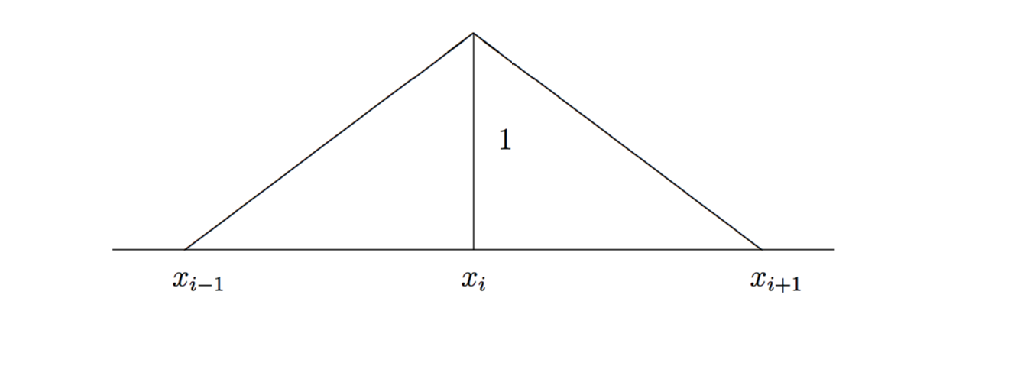# Finite Element Method

•Typical domain is$D=(0,1).$
• Let$h$ be the grid size.
• Let$\phi_i$ be basis functions on the grid.
•$V_h$ space of piecewise linear polynomials.
• Use a Galerkin projection onto the space.

In terms of the Finite Element space equation (1) takes the following form,${A(u_h(y),v_h)=l(v_h), \forall v_h \in V_h.$

If we furthermore express$u_h(y)$ as a linear combination of our basis functions we obtain,$(u_h(y))(x)=\sum^N_{i=1}U_{i}(y)\phi_{i}(x), U_i(y)\in \mathbb{R} ,$

we rewrite the above equation as,$\sum_{i=1}^{N_h}A(\phi_i,\phi_j)U_i(y)&=l(\phi_j), \text{ for } j=1,\ldots,N. \quad (2)$